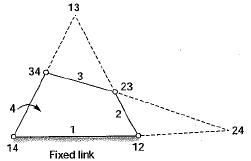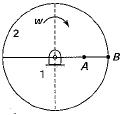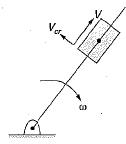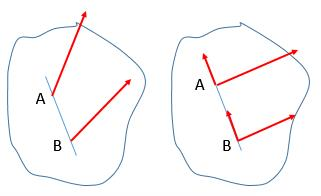# Velocity And Acceleration Analysis - 2

## 10 Questions MCQ Test Topicwise Question Bank for GATE Mechanical Engineering | Velocity And Acceleration Analysis - 2

Description
This mock test of Velocity And Acceleration Analysis - 2 for Mechanical Engineering helps you for every Mechanical Engineering entrance exam. This contains 10 Multiple Choice Questions for Mechanical Engineering Velocity And Acceleration Analysis - 2 (mcq) to study with solutions a complete question bank. The solved questions answers in this Velocity And Acceleration Analysis - 2 quiz give you a good mix of easy questions and tough questions. Mechanical Engineering students definitely take this Velocity And Acceleration Analysis - 2 exercise for a better result in the exam. You can find other Velocity And Acceleration Analysis - 2 extra questions, long questions & short questions for Mechanical Engineering on EduRev as well by searching above.
QUESTION: 1

Solution:
QUESTION: 2

Solution:
QUESTION: 3

### Consider a pin jointed 4-bar mechanism as shown in the figure below.Which of the following are permanent instantaneous centers?

Solution:

I12 and I14 are fixed instantaneous center.
I23 and I34 are permanent instantaneous center.
I13 and I24 are movable instantaneous center.

QUESTION: 4

A crank of radius 15 cm is rotating at 50 rpm with an angular acceleration of 60 rad/sec2. The tangential acceleration of the crank is about

Solution:

at = αr = 60 x 0.15 - 9 m/s

QUESTION: 5

Two points A and B located along the radius of wheel as shown below, have velocities of 80 and 140 m/s respectively.​The distance between the points A and B is 300 mm. The radius of the wheel (in mm) is

Solution:
QUESTION: 6

The magnitude of the Coriolis component of acceleration of a slider moving at a velocity V on a link rotating at angular speed ω is

Solution:

Magnitude of Coriolis component = 2 \/ω

QUESTION: 7

Klein’s construction can be used when

Solution:

Klein’s construction is used to determine the acceleration of various parts and this method is used when crank has uniform angular velocity.

QUESTION: 8

Coriolis component of acceleration is always

Solution:Coriolis component of acceleration appears in the direction perpendicular to the link.

QUESTION: 9

A constrained kinematic chain (mechanism) has n number of links. What is the number of instantaneous centres

Solution:
QUESTION: 10

There are two points P and Q on a planar rigid body. The relative velocity between the two points

Solution:

Given any two points A and B on a rigid body, let line AB be passing through points A and B, then the components of velocity of A and B along the line AB are always equal as the distance between A and B never changes or we can say relative velocity along AB is always zero.

Hence the relative velocity of the points must be perpendicular to the line joining them.